Markets
Futures
Earn
More
Cancel
FAQAccount FunctionsTutorialBinance Fan TokenBinance EarnCrypto Deposit/Withdrawal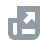Crypto Derivatives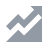FinanceAPISecurityOther Topics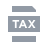Tax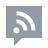Feed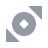Binance Convert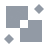NFTVIP
Home
Support Center
FAQ
Crypto Derivatives
Futures Contracts
USDⓈ-M Futures Contracts
How to Calculate Liquidation Price of USDⓈ-M Futures Contracts

# How to Calculate Liquidation Price of USDⓈ-M Futures Contracts

2020-08-14 02:14
Below is the liquidation price formula for USDⓈ-M futures contracts under the cross margin mode:
where:
Note:
• In Cross margin mode, WB is crossWalletBalance.
• In Isolated margin mode, WB is isolatedWalletBalance of the isolated position, TMM=0, UPNL=0, substitute the position quantity, MMR, cum into the formula to calculate.

## Maintenance Margin Rate

You can find the “Maintenance Margin Rate” from the table below with the position value in USDT.
For example, if the position size of a USDT-margined BTCUSDT contract is 264,000 USDT, then the maintenance margin rate would be 1% (or 0.01).
Note:
• If there is a discrepancy between the position notional (determined by the liquidation price calculation) and the assumed margin level* (The assumed margin level indicates the tier in which the position notional value may be classified), you must use the maintenance margin rate and the amount corresponding to the other margin levels* to recalculate the liquidation price.
• *contract 1
contract 1 is the specific symbol for which the liquidation price is being calculated
• * assumed margin level
• The assumed margin level indicates the tier in which the position notional value may be classified
• *other margin levels
• Other margin levels refer to margin tiers that are not the same as the assumed margin level. Margin tiers are listed on the Leverage & Margin page.

## Maintenance Amount

You can find the “Maintenance Amount” from the table below with the position value in USDT. For example, if the position size of a USDT-margined BTCUSDT contract is 500,000 USDT, then the maintenance amount would be 1,300 USDT.

## Maintenance Margin

Maintenance Margin = Position Notional * Maintenance Margin Rate on the level of position notional - Maintenance Amount on the level of position notional
For example, you have 10 BTCUSDT perpetual positions, and the latest mark price is 26,000. The position value is calculated as 10 * 26,000 = 260,000, which is located in the margin level 3. Thus, we can know the corresponding Maintenance Margin Rate and Maintenance Amount and substitute in the above formula:
Maintenance Margin = 10 * 26,000 * 1% - 1,300 = 1,300 USDT
All maintenance margin rates and amounts of USDⓈ-M futures contracts are available on the Leverage & Margin page.

## Example: Calculating Liquidation Price in One-way & Cross Margin mode

(WB) Wallet Balance = 1,535,443.01
You may find your maintenance margin rates and amounts through the tables above, as highlighted in the following image.
For ETHUSDT:
Taking into account that Maintenance margin = Notional value * Maintenance Margin rate-cum
Notional Value = Price * Size
Maintenance Margin of ETHUSDT = 356,512.508 (4918775.081 * 10.00% - 135365).
(WB) Wallet Balance = 1,535,443.01
(TMM1) Maintenance Margin of all other contracts, excluding Contract 1 = 71200.81144
(UPNL1) Unrealized PNL of all other contracts, excluding Contract 1 = -56,354.57
(cumB) Maintenance Amount of BOTH position (one-way mode) = 135,365.00
(cumL) Maintenance amount of LONG position (hedge mode) = 0
(cumS) Maintenance amount of SHORT position (hedge mode) = 0
(Side1BOTH) Direction of BOTH position, 1 as long position, -1 as short position = 1
(Position1BOTH) Absolute value of BOTH position size (one-way mode) = 3,683.979
(EP1BOTH) Entry Price of BOTH position (one-way mode) =1,456.84
(Position1LONG) Absolute value of LONG position size (hedge mode) = 0
(EP1LONG) Entry Price of LONG position (hedge mode) = 0
(Position1SHORT) Absolute value of SHORT position size (hedge mode) = 0
(EP1SHORT) Entry Price of SHORT position (hedge mode) = 0
(MMRB) Maintenance margin rate of BOTH position (one-way mode) = 10%
(MMRL) Maintenance margin rate of LONG position (hedge mode) = 0
(MMRS) Maintenance margin rate of SHORT position (hedge mode) = 0

### For BTCUSDT:

Taking into account that Maintenance margin=Notional value*Maintenance Margin rate-cum
Notional Value = Price * Size
Maintenance Margin of BTCUSDT = 71200.81144 (3500032.458 * 2.50% - 16300).
(WB) Wallet Balance = 1,535,443.01
(TMM1) Maintenance Margin of all other contracts, excluding Contract 1 = 356,512.508
(UPNL1) Unrealized PNL of all other contracts, excluding Contract 1 = -448,192.89
(cumB) Maintenance Amount of BOTH position (one-way mode) = 16,300.000
(cumL) Maintenance amount of LONG position (hedge mode) = 0.
(cumS) Maintenance amount of SHORT position (hedge mode) = 0
(Side1BOTH) Direction of BOTH position, 1 as long position, -1 as short position = 1
(Position1BOTH) Absolute value of BOTH position size (one-way mode) = 109.488
(EP1BOTH) Entry Price of BOTH position (one-way mode) =32,481.980
(Position1LONG) Absolute value of LONG position size (hedge mode) = 0
(EP1LONG) Entry Price of LONG position (hedge mode) = 0
(Position1SHORT) Absolute value of SHORT position size (hedge mode) = 0
(EP1SHORT) Entry Price of SHORT position (hedge mode) = 0
(MMRB) Maintenance margin rate of BOTH position (one-way mode) = 2.50%
(MMRL) Maintenance margin rate of LONG position (hedge mode) = 0
(MMRS) Maintenance margin rate of SHORT position (hedge mode) = 0

### Liquidation Price Formula:

With this information and by inputting the appropriate information in the formula we can see that the Liquidation Price for the ETHUSDT contract = 1153.26 and for BTCUSDT =26,316.89
Please note that there may be a slight variation due to decimals.